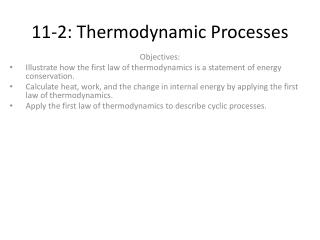Download Presentation11-2: Thermodynamic Processes11-2: Thermodynamic Processes - PowerPoint PPT Presentation

Download Presentation11-2: Thermodynamic Processes
An Image/Link below is provided (as is) to download presentation

Download Policy: Content on the Website is provided to you AS IS for your information and personal use and may not be sold / licensed / shared on other websites without getting consent from its author. While downloading, if for some reason you are not able to download a presentation, the publisher may have deleted the file from their server.

- - - - - - - - - - - - - - - - - - - - - - - - - - - E N D - - - - - - - - - - - - - - - - - - - - - - - - - - -
Presentation Transcript

1. 11-2: Thermodynamic Processes Objectives: Illustrate how the first law of thermodynamics is a statement of energy conservation. Calculate heat, work, and the change in internal energy by applying the first law of thermodynamics. Apply the first law of thermodynamics to describe cyclic processes.

2. THE FIRST LAW OF THERMODYNAMICS • Imagine a roller coaster that operates without friction. The car is raised against the force of gravity by work. • Once the car is freely moving, it will have a certain kinetic energy (KE) and a certain potential energy (PE). • The coaster will move slower (have a small KE) at the top of the rise (have a large PE) and faster (have a larger KE) at low points in the track (have a smaller PE). • However, the mechanical energy, KE + PE, remains constant throughout the ride’s duration.

3. THE FIRST LAW OF THERMODYNAMICS • If friction is taken into account, mechanical energy is not conserved • A steady decrease in the car’s total mechanical energy occurs because of work being done against the friction between the car’s axles and its bearings and between the car’s wheels and the coaster track. • Thus, the roller coaster’s internal energy increases by an amount equal to the decrease in the mechanical energy. • Most of this energy is then gradually dissipated to the air surrounding the roller coaster as heat and sound. If the internal energy for the roller coaster (the system) and the energy dissipated to the surrounding air (the environment) are taken into account, then the total energy will be constant.

4. THE FIRST LAW OF THERMODYNAMICS • The principle of energy conservation that takes into account a system’s internal energy as well as work and heat is called the first law of thermodynamics.

5. THE FIRST LAW OF THERMODYNAMICS • ∆U = Q − W • Change in system’s internal energy = (energy transferred to or from system as heat) − (energy transferred to or from system as work) • When this equation is used, all quantities must have the same energy units. • the SI unit for energy, the joule, will be used. • The thermodynamic processes discussed last section can therefore be expressed using the equation for the first law of thermodynamics.

6. A total of 135 J of work is done on a gaseous refrigerant as it undergoes compression. If the internal energy of the gas increases by 114 J during the process, what is the total amount of energy transferred as heat? Has energy been added to or removed from the refrigerant as heat? • Given: W = −135 J ∆U = 114 J • Find: Q = ? • ∆U = Q − W, so Q= ∆U+W Q= (114J) + (-135J) = −21 J

7. Cyclic Process • a thermodynamic process in which a system returns to the same conditions under which it started • In a refrigerator, the net amount of work done during each complete cycle must equal the net amount of energy transferred as heat to and from the refrigerant. • Energy is transferred as heat from the cold interior of the refrigerator to the even colder evaporating refrigerant (Qcold , or Qc). Energy is also transferred as heat from the hot condensing refrigerant to the relatively cooler air outside the refrigerator (Qhot , or Qh). • Therefore, the difference between Qhand Qcequals the net energy transferred as heat—and thus the net work done—during one cycle of the refrigeration process. • Wnet=Qh−Qc, where Qh>Qc

8. Heat engines use heat to do work • A refrigerator uses mechanical work to create a difference in temperature and thus transfers energy as heat. A heat engine is a device that does the opposite. It uses heat to do mechanical work. • Consider an internal combustion engine. It will burn fuel (chemical energy) to increase the amount of energy(heat) in a cylinder. • This increase in energy will create an increase in volume and push the piston down. • This heat is transferred to the cylinder and then to coolant in which it is released into the environment. • This linear motion is then converted to rotational motion to move the vehicle.